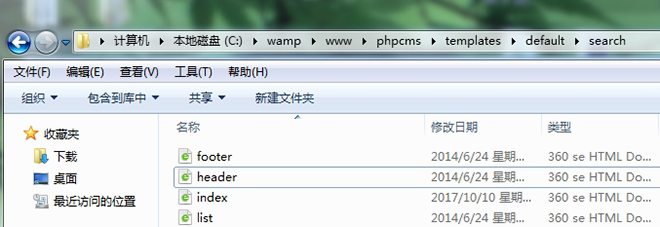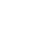# phpcms如何实现全站搜索功能

1. <form name="search_form1" target="_blank" onsubmit="return go(this)">
2. <input
3.  name="q" id="q" size="30" value="请输入关键字" onMouseOver="this.focus()"
4. onBlur="if (value ==''){value='请输入关键字'}" onFocus="this.select()"
5. onClick="if(this.value=='请输入关键字')this.value=''">
6. <INPUT name="word" type="hidden" value=0>
7. <INPUT name="myselectvalue" type="hidden" value=0>
8. <INPUT name="tn" type="hidden" value="sayyes">
9. <INPUT name="cl" type="hidden" value="3">
10. <input type="hidden" name="m" value="search"/>
11. <input type="hidden" name="c" value="index"/>
12. <input type="hidden" name="a" value="init"/>
13. <input type="hidden" name="typeid" value="1"/>
14. <input type="hidden" name="siteid" value="1"/>
15. <INPUT CHECKED name="myselect" onclick=javascriptthis.form.myselectvalue.value=0type=radio value=0>
16. <FONT color=#0000cc style="FONT-SIZE: 12px">百度搜索</FONT>
18. <FONT color=#ff0000 style="FONT-SIZE: 12px">站内</FONT>
19. <input type="submit" value="搜索">
20. </form>
21. <SCRIPT language=javascript>
22. <!--
23. function go(formname)
24. {
25. var url = "https://www.baidu.com/baidu";
26. formname.method = "get";
27. if (formname.myselectvalue.value == "1") {
28. url="{APP_PATH}index.php";
29. }
30. documentdocument.search_form1.word.value = document.search_form1.q.value;
31. formname.action = url;
32. return true;
33. }
34. //-->
35. </SCRIPT>

phpcms自带的搜索代码在默认模板的搜索模块里的index.html页面里，路径：/phpcms/templates/default/search/,代码如下：

``````<form name="search" type="get">
<input type="hidden" name="m" value="search"/>
<input type="hidden" name="c" value="index"/>
<input type="hidden" name="a" value="init"/>
<input type="hidden" name="typeid" value="{\$typeid}" id="typeid"/>
<input type="hidden" name="siteid" value="{\$siteid}" id="siteid"/>
<div class="sr_frm">
<div class="sr_frm_box">
<div class="sr_frmipt">
<input type="text" name="q" id="q" class="ipt">
<div class="sp" id="aca">▼</div>
<input type="submit" class="ss_btn" value="搜 索">
</div>
</div>
<div id="sr_infos" class="wrap sr_infoul"> </div>
</div>
</form> ``````2018年10月23日补充：动态网站纯静态后可能会遇到只有在首页和搜索列表页搜索功能才能正常使用的情况，解决方法戳“phpcms网站静态化后全站搜索功能不能正常使用如何解决”。•东方星雨
•deanhan
•1264822519
•aini3311
•a1051020101
•weiyiqi